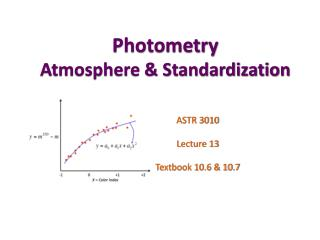DownloadDownload PresentationPhotometry Atmosphere & Standardization

# Photometry Atmosphere & Standardization

Download Presentation## Photometry Atmosphere & Standardization

- - - - - - - - - - - - - - - - - - - - - - - - - - - E N D - - - - - - - - - - - - - - - - - - - - - - - - - - -
##### Presentation Transcript

1. PhotometryAtmosphere & Standardization ASTR 3010 Lecture 13 Textbook 10.6 & 10.7

2. Extinction by Atmosphere • Observing the incoming radiation at depth H in the atmosphere. Measured spectrum φA(λ) where optical depth τ and X is air mass.

3. Different notations

4. Bouguer’s Law Take multiple measurements of non-varying object at several different airmasses! • one can get a mean extinction coeff from the slope • with known airmass, one can recover mλ for any other stars! slope = k X 0 1 2 3

5. Sources of extinction • Rayleigh scattering • Absorption by Ozone • Scattering by Aerosols • Molecular-band absorption stable over long time variable due to a weather system

6. Photometric Condition To be able to use Bouguer’s Law, we need two conditions • k is stationary • k is isotropic when these two conditions are met, the night is called “photometric” slope = k Example of non-stationary extinction during the obs. X 0 1 2 3

7. Measuring monochromatic extinction • Assume  use observatory’s value • Use a reference  observe a star with known mλ • From the Bouguer line of your measurements • Variable extinction / multi-night data • measure two standard stars at a given time at different airmass • repeat the pair observation several times per night • Use all data X 0 1 2 3

8. Heterochromatic extinction • Apparent magnitudes versus airmassdifferent slopes for different colors Forbes Effect = spectrum of a star changes with airmass

9. 2nd order extinction coefficients • Taylor Expand kP (or parameterize kP) • For example, (B-V) color can be used to indicate the spectral shape. • This color-dependent term is not changing rapidly and takes many data to measure one can use observatory’s value

10. Transformation to a standard system • instrumental (outside the atmosphere) magnitudes measured with two filters at λ1 and λ2 where standard wavelengths are λS1 and λS2. From we get Then, efficiency term zero-point constant color term color coefficient

11. Transformation to a standard system • In practice, you measure mλ1 and (color index)12 or mλ1 and mλ2 then plot -1 0 +1 +2 X = Color Index

12. Example (Homework) An observer used B and V filters to obtain four exposures of the same field at different air masses: two B exposures at air masses 1.05 and 2.13, and two V exposures at airmasses 1.10 and 2.48. Four stars in this field are photometric standards. Their measured magnitudes are given below.

13. Example (Homework) • Calculate extinction coefficients for the instrumental system for B and V bands. • Compute the standard transformation coefficients αV and αB-V (orαB) • Calculate standard magnitudes of Obj1 (i.e., V and B-V) whose instrumental magnitudes are v=9.850 and b=10.899 taken at airmass=1.50

14. Example (Homework) • Calculate extinction coefficients for the instrumental system for B and V bands. unknown unknown Plot b(2)-b(1)/(X2-X1) and measure the slope for k1(B-V)

15. Example (Homework) • Compute the standard transformation coefficients αV and αB-V (orαB) • Plot as a function of color index (e.g., B-V) • Slope = α12 • y-intercept = α1

16. Example (Homework) • Calculate standard magnitudes of Obj1 (i.e., V and B-V) whose instrumental magnitudes are v=9.850 and b=10.899 taken at airmass=1.50

17. In summary… Important Concepts Important Terms Extinction coefficient Forbes effect • Bouguer’s Law • Photometric condition • Standard Transformation • Chapter/sections covered in this lecture : 10.6 & 10.7